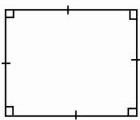HOME MATH DICTIONARY DOWNLOAD FEEDBACK DISCLAIMER
 Question: What is Square ? Answer: A square is the most symmetric quadrilateral. All its sides are of equal length and its angles are all right angles. Its diagonals are equal in length, bisect each other, and cross at right angles. It has 4 lines of symmetry and rotational symmetry of order 4. Its opposite sides are parallel. The square shape is commonly seen as a wall tile, or paving slab, or as the basic shape in a patchwork quilt, because it easily tessellates.Example of a Square: